# 机器学习 | 卷积神经网络详解 (二)——自己手写一个卷积神经网络# 一、卷积神经网络的前向传播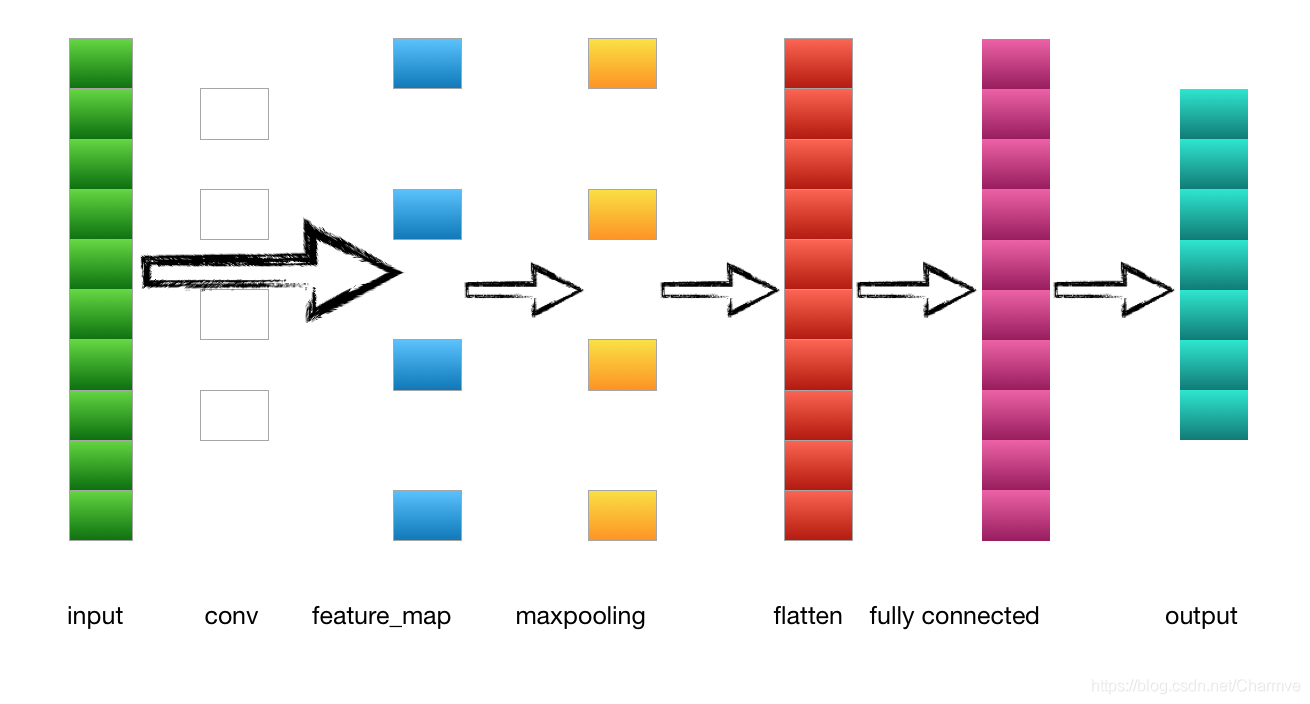## 1.输入层---->卷积层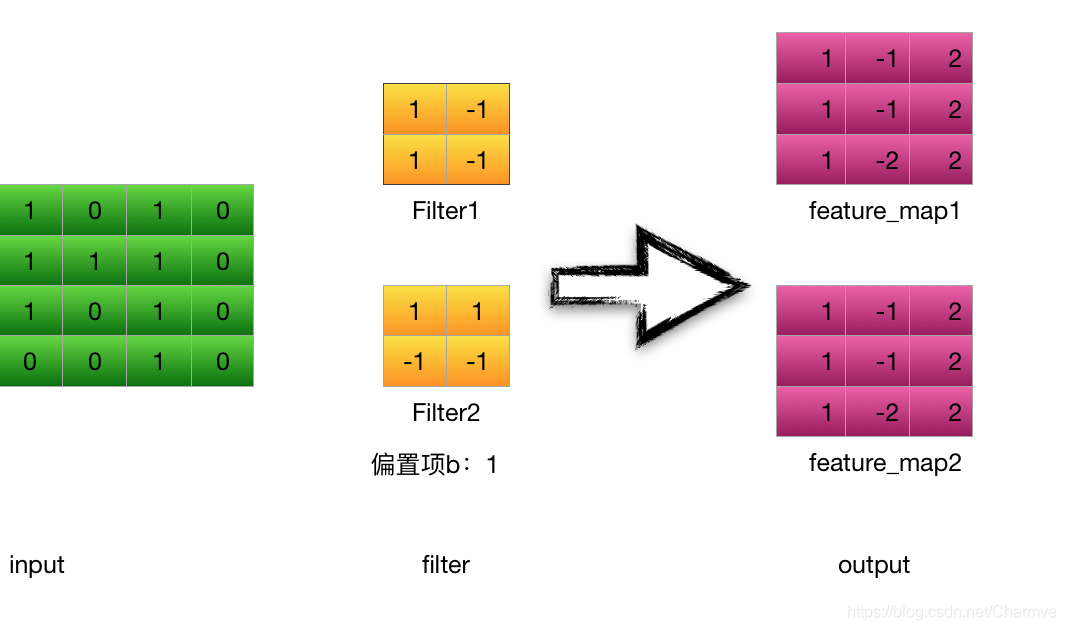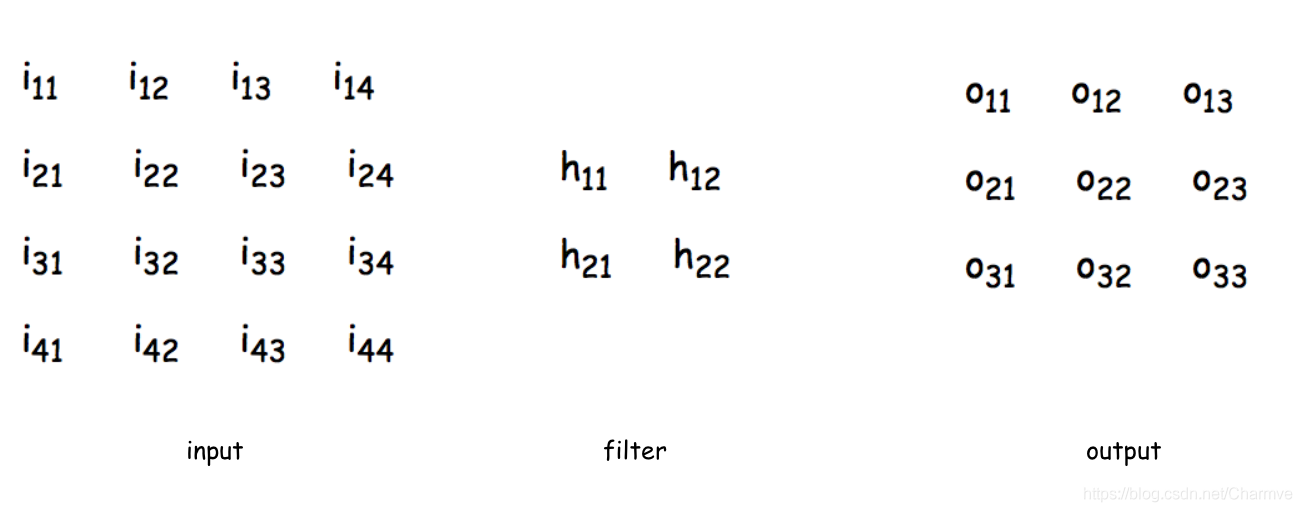## 2.卷积层---->池化层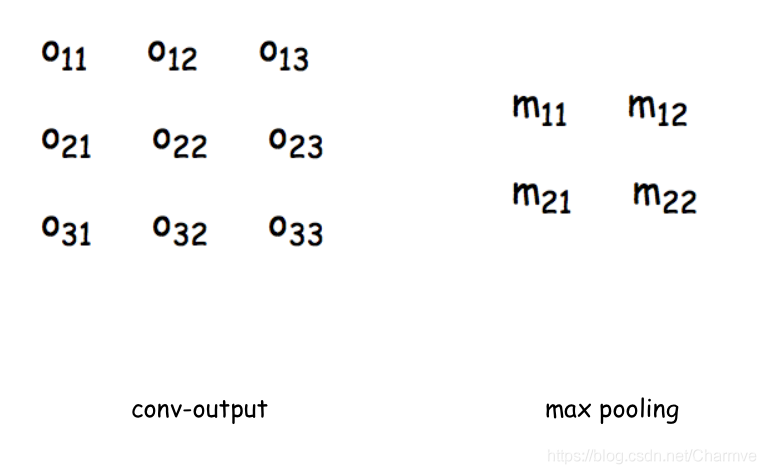# 二、卷积神经网络的反向传播

1. 通过前向传播计算每一层的输入值(如卷积后的 feature_map 的第一个神经元的输入：

1. 反向传播计算每个神经元的误差项​，其中 E 为损失函数计算得到的总体误差，可以用平方差，交叉熵等表示。

2. 计算每个神经元权重的梯度，

3. 更新权重(其中λ为学习率)

## 1. 卷积层的反向传播

每一个神经元的值都是上一个神经元的输入作为这个神经元的输入，经过激活函数激活之后输出，作为下一个神经元的输入，在这里我用 i_{11}i11​表示前一层,o_{11}o11​表示 i_{11}i11​的下一层。那么 net_{i_{11}}neti11​​就是 i_{11}i11​这个神经元的输入，net_{i_{11}}neti11​​就是 i_{11}i11​这个神经元的输出，同理，net_{o_{11}}neto11​​就是 o_{11}o11​这个神经元的输入，out_{o_{11}}outo11​​就是 o_{11}o11​这个神经元的输出,因为上一层神经元的输出 = 下一层神经元的输入，所以 out_{i_{11}}= net_{o_{11}}outi11​​=neto11​​，这里我为了简化，直接把 out_{i_{11}}outi11​​记为 i_{11}i11​

net_{i_{11}}neti11​​表示上一层的输入，out_{i_{11}}outi11​​表示上一层的输出

​的偏导：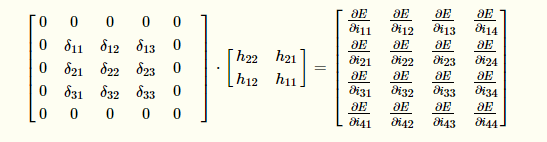## 2. 池化层的反向传播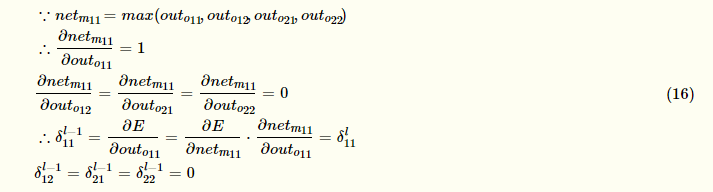# 三、手写一个卷积神经网络

## 1.定义一个卷积层

class ConvLayer(object):    '''    参数含义：    input_width:输入图片尺寸——宽度    input_height:输入图片尺寸——长度    channel_number:通道数，彩色为3，灰色为1    filter_width:卷积核的宽    filter_height:卷积核的长    filter_number:卷积核数量    zero_padding：补零长度    stride:步长    activator:激活函数    learning_rate:学习率    '''    def __init__(self, input_width, input_height,                 channel_number, filter_width,                 filter_height, filter_number,                 zero_padding, stride, activator,                 learning_rate):        self.input_width = input_width        self.input_height = input_height        self.channel_number = channel_number        self.filter_width = filter_width        self.filter_height = filter_height        self.filter_number = filter_number        self.zero_padding = zero_padding        self.stride = stride        self.output_width = \            ConvLayer.calculate_output_size(            self.input_width, filter_width, zero_padding,            stride)        self.output_height = \            ConvLayer.calculate_output_size(            self.input_height, filter_height, zero_padding,            stride)        self.output_array = np.zeros((self.filter_number,            self.output_height, self.output_width))        self.filters = []        for i in range(filter_number):            self.filters.append(Filter(filter_width,                filter_height, self.channel_number))        self.activator = activator        self.learning_rate = learning_rate

@staticmethod     def calculate_output_size(input_size,            filter_size, zero_padding, stride):         return (input_size - filter_size +             2 * zero_padding) / stride + 1

## 2.构造一个激活函数

class ReluActivator(object):    def forward(self, weighted_input):        #return weighted_input        return max(0, weighted_input)    def backward(self, output):        return 1 if output > 0 else 0

class SigmoidActivator(object):    def forward(self, weighted_input):        return 1.0 / (1.0 + np.exp(-weighted_input))    #the partial of sigmoid    def backward(self, output):        return output * (1 - output)

## 3.定义一个类，保存卷积层的参数和梯度

class Filter(object):    def __init__(self, width, height, depth):        #初始权重        self.weights = np.random.uniform(-1e-4, 1e-4,            (depth, height, width))        #初始偏置        self.bias = 0        self.weights_grad = np.zeros(            self.weights.shape)        self.bias_grad = 0    def __repr__(self):        return 'filter weights:\n%s\nbias:\n%s' % (            repr(self.weights), repr(self.bias))    def get_weights(self):        return self.weights    def get_bias(self):        return self.bias    def update(self, learning_rate):        self.weights -= learning_rate * self.weights_grad        self.bias -= learning_rate * self.bias_grad

## 4.卷积层的前向传播

### 1).获取卷积区域

# 获取卷积区域def get_patch(input_array, i, j, filter_width,              filter_height, stride):    '''    从输入数组中获取本次卷积的区域，    自动适配输入为2D和3D的情况    '''    start_i = i * stride    start_j = j * stride    if input_array.ndim == 2:        input_array_conv = input_array[            start_i : start_i + filter_height,            start_j : start_j + filter_width]        print "input_array_conv:",input_array_conv        return input_array_conv    elif input_array.ndim == 3:        input_array_conv = input_array[:,            start_i : start_i + filter_height,            start_j : start_j + filter_width]        print "input_array_conv:",input_array_conv        return input_array_conv

### 2).进行卷积运算

def conv(input_array,         kernel_array,         output_array,         stride, bias):    '''    计算卷积，自动适配输入为2D和3D的情况    '''    channel_number = input_array.ndim    output_width = output_array.shape    output_height = output_array.shape    kernel_width = kernel_array.shape[-1]    kernel_height = kernel_array.shape[-2]    for i in range(output_height):        for j in range(output_width):            output_array[i][j] = (                get_patch(input_array, i, j, kernel_width,                    kernel_height, stride) * kernel_array                ).sum() + bias

#增加Zero paddingdef padding(input_array, zp):    '''    为数组增加Zero padding，自动适配输入为2D和3D的情况    '''    if zp == 0:        return input_array    else:        if input_array.ndim == 3:            input_width = input_array.shape            input_height = input_array.shape            input_depth = input_array.shape            padded_array = np.zeros((                input_depth,                input_height + 2 * zp,                input_width + 2 * zp))            padded_array[:,                zp : zp + input_height,                zp : zp + input_width] = input_array            return padded_array        elif input_array.ndim == 2:            input_width = input_array.shape            input_height = input_array.shape            padded_array = np.zeros((                input_height + 2 * zp,                input_width + 2 * zp))            padded_array[zp : zp + input_height,                zp : zp + input_width] = input_array            return padded_array

### 4).进行前向传播

def forward(self, input_array):        '''        计算卷积层的输出        输出结果保存在self.output_array        '''        self.input_array = input_array        self.padded_input_array = padding(input_array,            self.zero_padding)        for f in range(self.filter_number):            filter = self.filters[f]            conv(self.padded_input_array,                filter.get_weights(), self.output_array[f],                self.stride, filter.get_bias())        element_wise_op(self.output_array,                        self.activator.forward)

# 对numpy数组进行element wise操作，将矩阵中的每个元素对应相乘def element_wise_op(array, op):    for i in np.nditer(array,                       op_flags=['readwrite']):        i[...] = op(i)

## 5.卷积层的反向传播

### 1).将误差传递到上一层

def bp_sensitivity_map(self, sensitivity_array,                           activator):        '''        计算传递到上一层的sensitivity map        sensitivity_array: 本层的sensitivity map        activator: 上一层的激活函数        '''        # 处理卷积步长，对原始sensitivity map进行扩展        expanded_array = self.expand_sensitivity_map(            sensitivity_array)        # full卷积，对sensitivitiy map进行zero padding        # 虽然原始输入的zero padding单元也会获得残差        # 但这个残差不需要继续向上传递，因此就不计算了        expanded_width = expanded_array.shape        zp = (self.input_width +              self.filter_width - 1 - expanded_width) / 2        padded_array = padding(expanded_array, zp)        # 初始化delta_array，用于保存传递到上一层的        # sensitivity map        self.delta_array = self.create_delta_array()        # 对于具有多个filter的卷积层来说，最终传递到上一层的        # sensitivity map相当于所有的filter的        # sensitivity map之和        for f in range(self.filter_number):            filter = self.filters[f]            # 将filter权重翻转180度            flipped_weights = np.array(map(                lambda i: np.rot90(i, 2),                filter.get_weights()))            # 计算与一个filter对应的delta_array            delta_array = self.create_delta_array()            for d in range(delta_array.shape):                conv(padded_array[f], flipped_weights[d],                    delta_array[d], 1, 0)            self.delta_array += delta_array        # 将计算结果与激活函数的偏导数做element-wise乘法操作        derivative_array = np.array(self.input_array)        element_wise_op(derivative_array,                        activator.backward)        self.delta_array *= derivative_array

### 2).保存传递到上一层的 sensitivity map 的数组

def create_delta_array(self):        return np.zeros((self.channel_number,            self.input_height, self.input_width))

### 3).计算代码梯度

def bp_gradient(self, sensitivity_array):        # 处理卷积步长，对原始sensitivity map进行扩展        expanded_array = self.expand_sensitivity_map(            sensitivity_array)        for f in range(self.filter_number):            # 计算每个权重的梯度            filter = self.filters[f]            for d in range(filter.weights.shape):                conv(self.padded_input_array[d],                     expanded_array[f],                     filter.weights_grad[d], 1, 0)            # 计算偏置项的梯度            filter.bias_grad = expanded_array[f].sum()

### 4).按照梯度下降法更新参数

def update(self):        '''        按照梯度下降，更新权重        '''        for filter in self.filters:            filter.update(self.learning_rate)

## 6.MaxPooling 层的训练

### 1).定义 MaxPooling 类

class MaxPoolingLayer(object):    def __init__(self, input_width, input_height,                 channel_number, filter_width,                 filter_height, stride):        self.input_width = input_width        self.input_height = input_height        self.channel_number = channel_number        self.filter_width = filter_width        self.filter_height = filter_height        self.stride = stride        self.output_width = (input_width -            filter_width) / self.stride + 1        self.output_height = (input_height -            filter_height) / self.stride + 1        self.output_array = np.zeros((self.channel_number,            self.output_height, self.output_width))

### 2).前向传播计算

# 前向传播    def forward(self, input_array):        for d in range(self.channel_number):            for i in range(self.output_height):                for j in range(self.output_width):                    self.output_array[d,i,j] = (                        get_patch(input_array[d], i, j,                            self.filter_width,                            self.filter_height,                            self.stride).max())

### 3).反向传播计算

#反向传播    def backward(self, input_array, sensitivity_array):        self.delta_array = np.zeros(input_array.shape)        for d in range(self.channel_number):            for i in range(self.output_height):                for j in range(self.output_width):                    patch_array = get_patch(                        input_array[d], i, j,                        self.filter_width,                        self.filter_height,                        self.stride)                    k, l = get_max_index(patch_array)                    self.delta_array[d,                        i * self.stride + k,                        j * self.stride + l] = \                        sensitivity_array[d,i,j]

#coding:utf-8'''Created by huxiaoman 2017.11.22'''import numpy as npfrom activators import ReluActivator,IdentityActivatorclass ConvLayer(object):    def __init__(self,input_width,input_weight,             channel_number,filter_width,             filter_height,filter_number,             zero_padding,stride,activator,             learning_rate):        self.input_width = input_width        self.input_height = input_height        self.channel_number = channel_number        self.filter_width = filter_width        self.filter_height = filter_height        self.filter_number = filter_number        self.zero_padding = zero_padding        self.stride = stride #此处可以加上stride_x, stride_y        self.output_width = ConvLayer.calculate_output_size(                self.input_width,filter_width,zero_padding,                stride)        self.output_height = ConvLayer.calculate_output_size(                self.input_height,filter_height,zero_padding,                stride)        self.output_array = np.zeros((self.filter_number,                self.output_height,self.output_width))        self.filters = []        for i in range(filter_number):                self.filters.append(Filter(filter_width,                filter_height,self.channel_number))        self.activator = activator        self.learning_rate = learning_rate    def forward(self,input_array):        '''        计算卷积层的输出        输出结果保存在self.output_array        '''        self.input_array = input_array        self.padded_input_array = padding(input_array,            self.zero_padding)        for i in range(self.filter_number):            filter = self.filters[f]            conv(self.padded_input_array,                 filter.get_weights(), self.output_array[f],                 self.stride, filter.get_bias())            element_wise_op(self.output_array,                    self.activator.forward)def get_batch(input_array, i, j, filter_width,filter_height,stride):    '''    从输入数组中获取本次卷积的区域，    自动适配输入为2D和3D的情况    '''    start_i = i * stride    start_j = j * stride    if input_array.ndim == 2:        return input_array[            start_i : start_i + filter_height,            start_j : start_j + filter_width]    elif input_array.ndim == 3:        return input_array[            start_i : start_i + filter_height,                        start_j : start_j + filter_width]# 获取一个2D区域的最大值所在的索引def get_max_index(array):    max_i = 0    max_j = 0    max_value = array[0,0]    for i in range(array.shape):        for j in range(array.shape):            if array[i,j] > max_value:                max_value = array[i,j]                max_i, max_j = i, j    return max_i, max_jdef conv(input_array,kernal_array,    output_array,stride,bias):    '''    计算卷积，自动适配输入2D,3D的情况    '''    channel_number = input_array.ndim    output_width = output_array.shape    output_height = output_array.shape    kernel_width = kernel_array.shape[-1]    kernel_height = kernel_array.shape[-2]    for i in range(output_height):        for j in range(output_width):            output_array[i][j] = (                get_patch(input_array, i, j, kernel_width,                    kernel_height,stride) * kernel_array).sum() +biasdef element_wise_op(array, op):    for i in np.nditer(array,               op_flags = ['readwrite']):        i[...] = op(i)class ReluActivators(object):    def forward(self, weighted_input):        # Relu计算公式 = max(0,input)        return max(0, weighted_input)    def backward(self,output):        return 1 if output > 0 else 0class SigmoidActivator(object):            def forward(self,weighted_input):        return 1 / (1 + math.exp(- weighted_input))        def backward(self,output):        return output * (1 - output)

def init_test():    a = np.array(        [[[0,1,1,0,2],          [2,2,2,2,1],          [1,0,0,2,0],          [0,1,1,0,0],          [1,2,0,0,2]],         [[1,0,2,2,0],          [0,0,0,2,0],          [1,2,1,2,1],          [1,0,0,0,0],          [1,2,1,1,1]],         [[2,1,2,0,0],          [1,0,0,1,0],          [0,2,1,0,1],          [0,1,2,2,2],          [2,1,0,0,1]]])    b = np.array(        [[[0,1,1],          [2,2,2],          [1,0,0]],         [[1,0,2],          [0,0,0],          [1,2,1]]])    cl = ConvLayer(5,5,3,3,3,2,1,2,IdentityActivator(),0.001)    cl.filters.weights = np.array(        [[[-1,1,0],          [0,1,0],          [0,1,1]],         [[-1,-1,0],          [0,0,0],          [0,-1,0]],         [[0,0,-1],          [0,1,0],          [1,-1,-1]]], dtype=np.float64)    cl.filters.bias=1    cl.filters.weights = np.array(        [[[1,1,-1],          [-1,-1,1],          [0,-1,1]],         [[0,1,0],         [-1,0,-1],          [-1,1,0]],         [[-1,0,0],          [-1,0,1],          [-1,0,0]]], dtype=np.float64)    return a, b, cl

def test():    a, b, cl = init_test()    cl.forward(a)    print "前向传播结果:", cl.output_array    cl.backward(a, b, IdentityActivator())    cl.update()    print "反向传播后更新得到的filter1:",cl.filters    print "反向传播后更新得到的filter2:",cl.filtersif __name__ == "__main__":        test()

前向传播结果: [[[ 6.  7.  5.]  [ 3. -1. -1.]  [ 2. -1.  4.]] [[ 2. -5. -8.]  [ 1. -4. -4.]  [ 0. -5. -5.]]]反向传播后更新得到的filter1: filter weights:array([[[-1.008,  0.99 , -0.009],        [-0.005,  0.994, -0.006],        [-0.006,  0.995,  0.996]],       [[-1.004, -1.001, -0.004],        [-0.01 , -0.009, -0.012],        [-0.002, -1.002, -0.002]],       [[-0.002, -0.002, -1.003],        [-0.005,  0.992, -0.005],        [ 0.993, -1.008, -1.007]]])bias:0.99099999999999999反向传播后更新得到的filter2: filter weights:array([[[  9.98000000e-01,   9.98000000e-01,  -1.00100000e+00],        [ -1.00400000e+00,  -1.00700000e+00,   9.97000000e-01],        [ -4.00000000e-03,  -1.00400000e+00,   9.98000000e-01]],       [[  0.00000000e+00,   9.99000000e-01,   0.00000000e+00],        [ -1.00900000e+00,  -5.00000000e-03,  -1.00400000e+00],        [ -1.00400000e+00,   1.00000000e+00,   0.00000000e+00]],       [[ -1.00400000e+00,  -6.00000000e-03,  -5.00000000e-03],        [ -1.00200000e+00,  -5.00000000e-03,   9.98000000e-01],        [ -1.00200000e+00,  -1.00000000e-03,   0.00000000e+00]]])bias:-0.0070000000000000001

conv_pool_1 = paddle.networks.simple_img_conv_pool(        input=img,        filter_size=5,        num_filters=20,        num_channel=1,        pool_size=2,        pool_stride=2,        act=paddle.activation.Relu())

conv = img_conv_layer(input=data, filter_size=1, filter_size_y=1,                              num_channels=8,                              num_filters=16, stride=1,                              bias_attr=False,                              act=ReluActivation())

def img_conv_layer(input,                   filter_size,                   num_filters,                   name=None,                   num_channels=None,                   act=None,                   groups=1,                   stride=1,                   padding=0,                   dilation=1,                   bias_attr=None,                   param_attr=None,                   shared_biases=True,                   layer_attr=None,                   filter_size_y=None,                   stride_y=None,                   padding_y=None,                   dilation_y=None,                   trans=False,                   layer_type=None):    """    适合图像的卷积层。Paddle可以支持正方形和长方形两种图片尺寸的输入        也可适用于图像的反卷积(Convolutional Transpose，即deconv)。    同样可支持正方形和长方形两种尺寸输入。    num_channel:输入图片的通道数。可以是1或者3，或者是上一层的通道数(卷积核数目 * 组的数量)    每一个组都会处理图片的一些通道。举个例子，如果一个输入如偏的num_channel是256，设置4个group，    32个卷积核，那么会创建32*4 = 128个卷积核来处理输入图片。通道会被分成四块，32个卷积核会先    处理64(256/4=64)个通道。剩下的卷积核组会处理剩下的通道。    name:层的名字。可选，自定义。    type:basestring    input:这个层的输入    type:LayerOutPut    filter_size:卷积核的x维，可以理解为width。                如果是正方形，可以直接输入一个元祖组表示图片的尺寸    type:int/ tuple/ list    filter_size_y:卷积核的y维，可以理解为height。                PaddlePaddle支持长方形的图片尺寸，所以卷积核的尺寸为(filter_size,filter_size_y)    type:int/ None    act: 激活函数类型。默认选Relu    type:BaseActivation    groups:卷积核的组数量    type:int        stride: 水平方向的滑动步长。或者世界输入一个元祖，代表水平数值滑动步长相同。    type:int/ tuple/ list    stride_y:垂直滑动步长。    type:int         padding: 补零的水平维度，也可以直接输入一个元祖，水平和垂直方向上补零的维度相同。    type:int/ tuple/ list    padding_y:垂直方向补零的维度    type:int    dilation:水平方向的扩展维度。同样可以输入一个元祖表示水平和初值上扩展维度相同    :type:int/ tuple/ list    dilation_y:垂直方向的扩展维度    type:int    bias_attr:偏置属性              False：不定义bias   True：bias初始化为0    type: ParameterAttribute/ None/ bool/ Any    num_channel：输入图片的通道channel。如果设置为None，自动生成为上层输出的通道数    type: int    param_attr:卷积参数属性。设置为None表示默认属性    param_attr:ParameterAttribute    shared_bias:设置偏置项是否会在卷积核中共享    type:bool    layer_attr: Layer的 Extra Attribute    type:ExtraLayerAttribute    param trans:如果是convTransLayer，设置为True，如果是convlayer设置为conv    type:bool    layer_type:明确layer_type，默认为None。               如果trans= True，必须是exconvt或者cudnn_convt，否则的话要么是exconv，要么是cudnn_conv               ps:如果是默认的话，paddle会自动选择适合cpu的ExpandConvLayer和适合GPU的CudnnConvLayer               当然，我们自己也可以明确选择哪种类型    type:string    return:LayerOutput object    rtype:LayerOutput    """def img_conv_layer(input,                   filter_size,                   num_filters,                   name=None,                   num_channels=None,                   act=None,                   groups=1,                   stride=1,                   padding=0,                   dilation=1,                   bias_attr=None,                   param_attr=None,                   shared_biases=True,                   layer_attr=None,                   filter_size_y=None,                   stride_y=None,                   padding_y=None,                   dilation_y=None,                   trans=False,                   layer_type=None):    if num_channels is None:        assert input.num_filters is not None        num_channels = input.num_filters    if filter_size_y is None:        if isinstance(filter_size, collections.Sequence):            assert len(filter_size) == 2            filter_size, filter_size_y = filter_size        else:            filter_size_y = filter_size    if stride_y is None:        if isinstance(stride, collections.Sequence):            assert len(stride) == 2            stride, stride_y = stride        else:            stride_y = stride    if padding_y is None:        if isinstance(padding, collections.Sequence):            assert len(padding) == 2            padding, padding_y = padding        else:            padding_y = padding    if dilation_y is None:        if isinstance(dilation, collections.Sequence):            assert len(dilation) == 2            dilation, dilation_y = dilation        else:            dilation_y = dilation    if param_attr.attr.get('initial_smart'):        # special initial for conv layers.        init_w = (2.0 / (filter_size**2 * num_channels))**0.5        param_attr.attr["initial_mean"] = 0.0        param_attr.attr["initial_std"] = init_w        param_attr.attr["initial_strategy"] = 0        param_attr.attr["initial_smart"] = False    if layer_type:        if dilation > 1 or dilation_y > 1:            assert layer_type in [                "cudnn_conv", "cudnn_convt", "exconv", "exconvt"            ]        if trans:            assert layer_type in ["exconvt", "cudnn_convt"]        else:            assert layer_type in ["exconv", "cudnn_conv"]        lt = layer_type    else:        lt = LayerType.CONVTRANS_LAYER if trans else LayerType.CONV_LAYER    l = Layer(        name=name,        inputs=Input(            input.name,            conv=Conv(                filter_size=filter_size,                padding=padding,                dilation=dilation,                stride=stride,                channels=num_channels,                groups=groups,                filter_size_y=filter_size_y,                padding_y=padding_y,                dilation_y=dilation_y,                stride_y=stride_y),            **param_attr.attr),        active_type=act.name,        num_filters=num_filters,        bias=ParamAttr.to_bias(bias_attr),        shared_biases=shared_biases,        type=lt,        **ExtraLayerAttribute.to_kwargs(layer_attr))    return LayerOutput(        name,        lt,        parents=[input],        activation=act,        num_filters=num_filters,        size=l.config.size)

• 支持长方形和正方形的图片尺寸

• 支持滑动步长 stride、补零 zero_padding、扩展 dilation 在水平和垂直方向上设置不同的值

• 支持偏置项卷积核中能够共享

• 自动适配 cpu 和 gpu 的卷积网络

# 总结

CNN 应用案例：△微信扫一扫关注「迈微电子研发社」公众号△扫码加入「迈微电子研发社」学习辅导群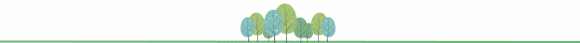## 评论